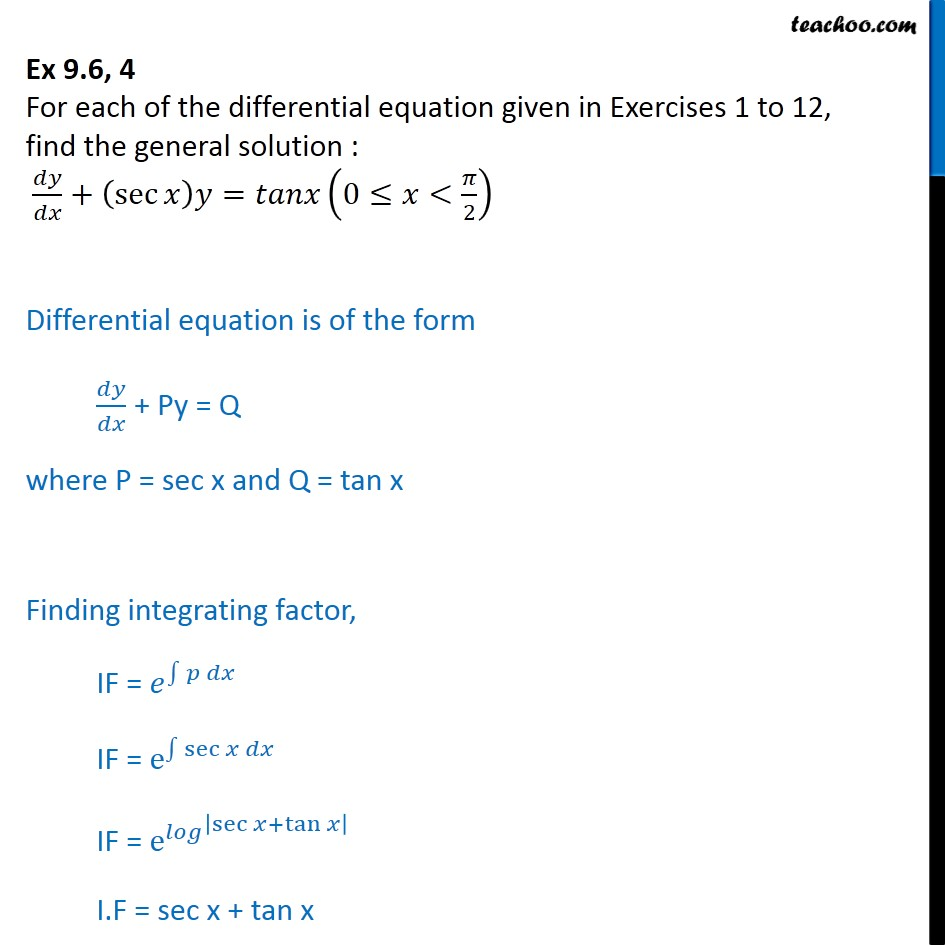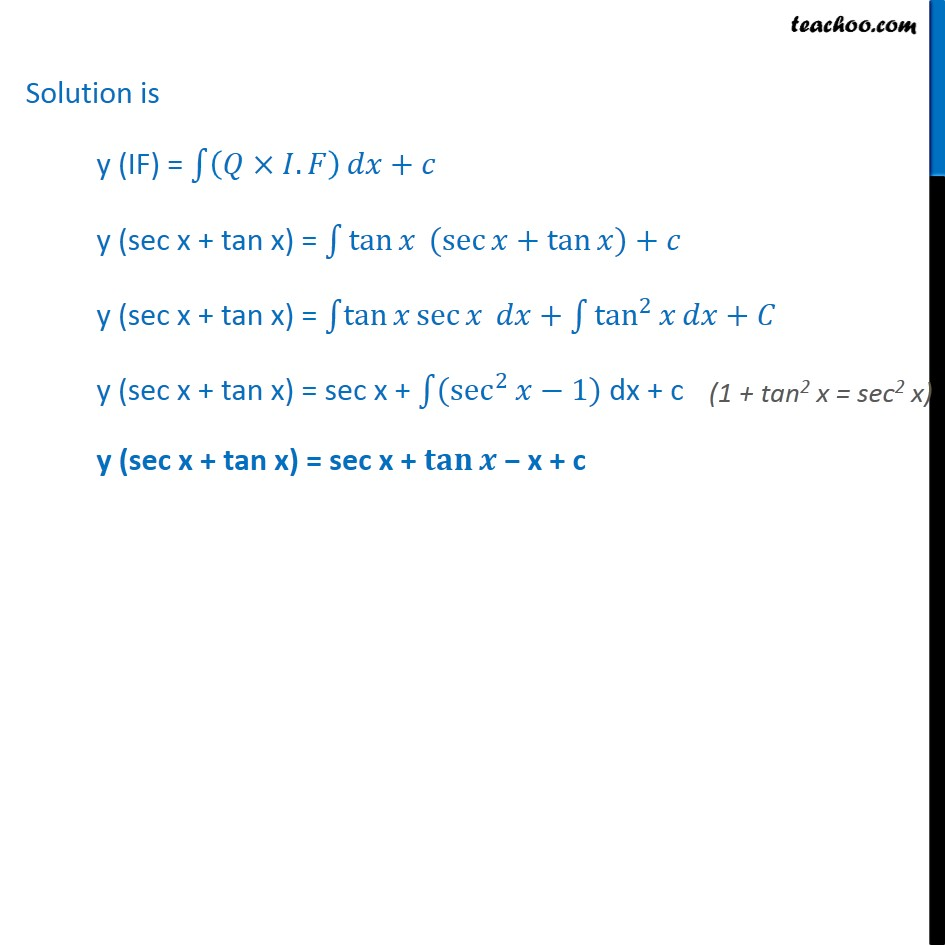Ex 9.5

Chapter 9 Class 12 Differential Equations
Serial order wiseLearn in your speed, with individual attention - Teachoo Maths 1-on-1 Class

### Transcript

Ex 9.5, 4 For each of the differential equation given in Exercises 1 to 12, find the general solution : + sec = 0 < 2 Differential equation is of the form + Py = Q where P = sec x and Q = tan x Finding integrating factor, IF = IF = e sec IF = e sec + tan I.F = sec x + tan x Solution is y (IF) = . + y (sec x + tan x) = tan ( sec + tan ) + y (sec x + tan x) = tan sec + tan 2 + y (sec x + tan x) = sec x + ( sec 2 1) dx + c y (sec x + tan x) = sec x + x + c